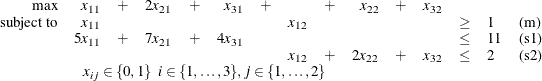## Getting Started: Decomposition Algorithm

This example illustrates how you can use the decomposition algorithm to solve a simple mixed integer linear program. Suppose you want to solve the following problem:It is obvious from the structure of the problem that if constraint (m) is removed, then the remaining constraints (s1) and (s2) decompose into two independent subproblems. The next two sections describe how to solve this MILP by using the decomposition algorithm in the OPTMODEL procedure and OPTMILP procedure, respectively.